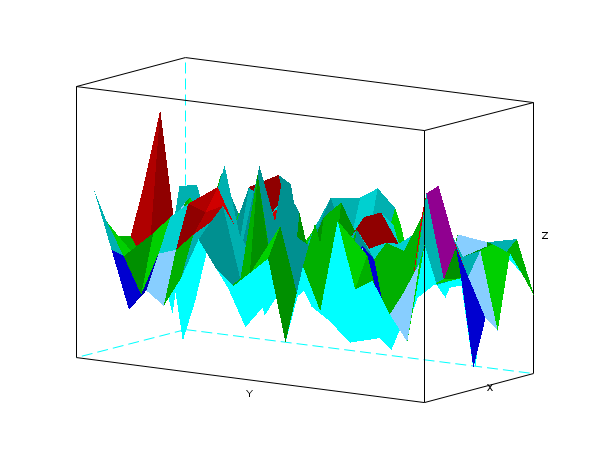Scilab Home page | Wiki | Bug tracker | Forge | Mailing list archives | ATOMS | File exchange
Change language to: English - Français - Português - 日本語 -

See the recommended documentation of this function

Справка Scilab >> Графики > 3d_plot > plot3d1

# plot3d1

3D gray or color level plot of a surface

### Calling Sequence

```plot3d1(x,y,z,[theta,alpha,leg,flag,ebox])
plot3d1(xf,yf,zf,[theta,alpha,leg,flag,ebox])
plot3d1(x,y,z,<opts_args>)
plot3d1(xf,yf,zf,<opts_args>)```

### Arguments

x,y

row vectors of sizes n1 and n2 (x-axis and y-axis coordinates). These coordinates must be monotone.

z

matrix of size (n1,n2). `z(i,j)` is the value of the surface at the point (x(i),y(j)).

xf,yf,zf

matrices of size (nf,n). They define the facets used to draw the surface. There are `n` facets. Each facet `i` is defined by a polygon with `nf` points. The x-axis, y-axis and z-axis coordinates of the points of the ith facet are given respectively by `xf(:,i)`, `yf(:,i)` and `zf(:,i)`.

<opt_args>

This represents a sequence of statements `key1=value1, key2=value2`,... where `key1`, `key2,...` can be one of the following: theta, alpha ,leg,flag,ebox (see definition below).

theta, alpha

real values giving in degree the spherical coordinates of the observation point.

leg

string defining the labels for each axis with @ as a field separator, for example "X@Y@Z".

flag

a real vector of size three. `flag=[mode,type,box]`.

mode

an integer (surface color).

mode>0

the surface is painted with color `"mode"` ; the boundary of the facet is drawn with current line style and color.

mode=0:

a mesh of the surface is drawn.

mode<0:

the surface is painted with color `"-mode"` ; the boundary of the facet is not drawn.

Note that the surface color treatement can be done using `color_mode` and `color_flag` options through the surface entity properties (see surface_properties).

type

an integer (scaling).

type=0:

the plot is made using the current 3D scaling (set by a previous call to `param3d`, `plot3d`, `contour` or `plot3d1`).

type=1:

rescales automatically 3d boxes with extreme aspect ratios, the boundaries are specified by the value of the optional argument `ebox`.

type=2:

rescales automatically 3d boxes with extreme aspect ratios, the boundaries are computed using the given data.

type=3:

3d isometric with box bounds given by optional `ebox`, similarily to `type=1`.

type=4:

3d isometric bounds derived from the data, to similarily`type=2`.

type=5:

3d expanded isometric bounds with box bounds given by optional `ebox`, similarily to `type=1`.

type=6:

3d expanded isometric bounds derived from the data, similarily to `type=2`.

Note that axes boundaries can be customized through the axes entity properties (see axes_properties).

box

an integer (frame around the plot).

box=0:

nothing is drawn around the plot.

box=1:

unimplemented (like box=0).

box=2:

only the axes behind the surface are drawn.

box=3:

a box surrounding the surface is drawn and captions are added.

box=4:

a box surrounding the surface is drawn, captions and axes are added.

Note that axes aspect can also be customized through the axes entity properties (see axes_properties).

ebox

It specifies the boundaries of the plot as the vector `[xmin,xmax,ymin,ymax,zmin,zmax]`. This argument is used together with `type` in `flag` : if it is set to `1`, `3` or `5` (see above to see the corresponding behaviour). If `flag` is missing, `ebox` is not taken into acoount.

Note that, when specified, the `ebox` argument acts on the `data_bounds` field that can also be reset through the axes entity properties (see axes_properties).

### Description

`plot3d1` plots a surface with colors depending on the z-level of the surface. This special plot function can also be enabled setting `color_flag=1` after a `plot3d` (see surface_properties)

Enter the command `plot3d1()` to see a demo.

### Examples

```// simple plot using z=f(x,y)
t=[0:0.3:2*%pi]';
z=sin(t)*cos(t');
plot3d1(t,t,z)``````// same plot using facets computed by genfac3d
t=[0:0.3:2*%pi]';
z=sin(t)*cos(t');
[xx,yy,zz]=genfac3d(t,t,z);
plot3d1(xx,yy,zz)``````// multiple plots
t=[0:0.3:2*%pi]';
z=sin(t)*cos(t');
[xx,yy,zz]=genfac3d(t,t,z);
plot3d1([xx xx],[yy yy],[zz 4+zz])``````// simple plot with viewpoint and captions
plot3d1(1:10,1:20,10*rand(10,20),35,45,"X@Y@Z",[2,2,3])``````// same plot without grid
plot3d1(1:10,1:20,10*rand(10,20),35,45,"X@Y@Z",[-2,2,3])``````// plot of a sphere using facets computed by eval3dp
deff("[x,y,z]=sph(alp,tet)",["x=r*cos(alp).*cos(tet)+orig(1)*ones(tet)";..
"y=r*cos(alp).*sin(tet)+orig(2)*ones(tet)";..
"z=r*sin(alp)+orig(3)*ones(tet)"]);
r=1; orig=[0 0 0];
[xx,yy,zz]=eval3dp(sph,linspace(-%pi/2,%pi/2,40),linspace(0,%pi*2,20));
plot3d(xx,yy,zz)
e=gce();
e.color_flag=1;
scf(2);
plot3d1(xx,yy,zz) // the 2 graphics are similar```• plot3d — 3D plot of a surface
• gca — Return handle of current axes.
• gce — Get current entity handle.
• scf — set the current graphic figure (window)
• clf — Clear or reset or reset a figure or a frame uicontrol.